# 10 Multiple Choice Calculus

## 10 Multiple Choice Calculus

I’m trying to study for my Calculus course and I need some help to understand this question.

1.

The graph of f ‘(x) is continuous, positive, and has a relative maximum at x = 0. Which of the following statements must be true? (5 points)

 The graph of f is always concave down. The graph of f is always increasing. The graph of f has a relative maximum at x = 0. The graph of f has a relative minimum at x = 0.

2.

Below is the graph of f ‘(x), the derivative of f(x), and has x-intercepts at x = -3, x = 1, and x = 2 and a relative maximum at x = -1.5 and a relative minimum at x = 1.5. Which of the following statement is false?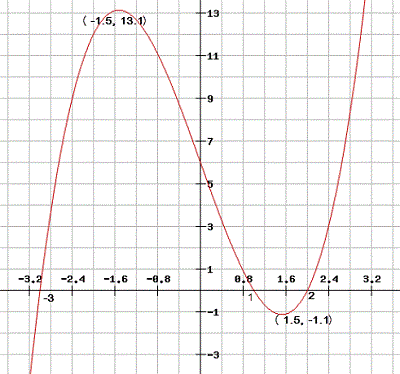(5 points)

 f is concave up from x = -1.5 to x = 1.5. f has an inflection point at x = 1.5. f has a relative minimum at x = 2. All of these are false.

3.

The graph of y = f ‘(x), the derivative of f(x), is shown below. List the intervals where the graph of f is concave down.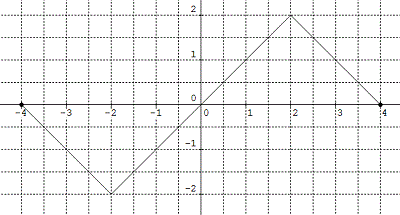(5 points)

 (-4, -2) U (2, 4) (-2, 2) (-4, 0) (0, 4)

4.

Which of the following functions grows the fastest as x grows without bound? (5 points)

 f(x) = ex g(x) = ecosx h(x) =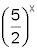They all grow at the same rate.

5.

Compare the growth rate of the functions f(x) = x3 + 1 and g(x) = x2 . (5 points)

 f(x) grows faster than g(x). g(x) grows faster than f(x). f(x) and g(x) grow at the same rate. It cannot be determined.

6.

f is a function that is differentiable for all reals. The value of f ‘(x) is given for several values of x in the table below.

x -8 -3 0 3 8
f ‘(x) -4 -2 0 4 5

If f ‘(x) is always increasing, which statement about f(x) must be true? (5 points)

 f(x) passes through the origin. f(x) is concave downwards for all x. f(x) has a relative minimum at x = 0. f(x) has a point of inflection at x = 0.

7.

f is a differentiable function on the interval [0, 1] and g(x) = f(2x). The table below gives values of f ‘(x). What is the value of g ‘(0.1)? (5 points)

x 0.1 0.2 0.3 0.4 0.5
f ‘(x) 1 2 3 -4 5

 1 2 4 Cannot be determined

8.

Use the graph of f(t) = 2t + 2 on the interval [-1, 4] to write the function F(x), where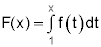. (5 points)

 F(x) = x2 + 3x F(x) = x2 + 2x – 12 F(x) = x2 + 2x – 3 F(x) = x2 + 4x – 8

9.

The velocity of a particle moving along the x-axis is v(t) = t2 + 2t + 1, with t measured in minutes and v(t) measured in feet per minute. To the nearest foot find the total distance travelled by the particle from t = 0 to t = 2 minutes. (5 points)

10.

Find the range of the function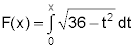. (5 points)

 [-6, 6] [0, 6] [0, 9π] [0, 18π]